# "I do not know what I may appear to the world, but to myself I seem to have been only a boy playing on the sea-shore, and diverting myself in now and then finding a smoother pebble or a prettier shell than ordinary, whilst the great ocean of truth lay all undiscovered before me."   Isaac Newton

"A mathematician, like a painter or a poet, is a maker of patterns. If his patterns are more permanent than theirs, it is because they are made with ideas."   G.H.Hardy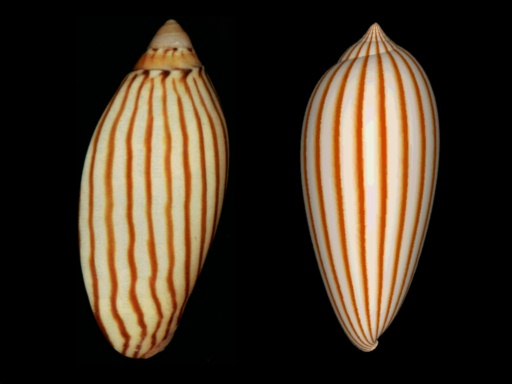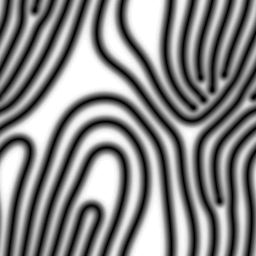My research areas are partial differential equations, dynamical systems and applications of biology, chemistry, physics. Partial differential equations (PDE) are traditional mathematical models for physics, chemistry and engineering problems based on the differential and integral calculus developed by Issac Newton . In a PDE model, physical quantities are assumed to be continuous and differentiable functions depending on several variables such as the time, spatial coordinates. Examples of such functions are concentration and density functions of chemical or biological organisms. The rates of change with respect to various variables and physical laws yield partial differential equations. In early years, PDEs are mostly used to model physical phenomena, such as heat equation, wave equation, Navier-Stokes equations in fluid mechanics (which is one of the Millennium Prize Problems). However the applications of PDEs now include biological sciences, economics, finances, and many other subjects requiring qualitative analysis.

My current interests are on the spatial-temporal models of biological and chemical systems. An important class of models is the reaction-diffusion equations based on the diffusion mechanism and reaction/interacting of different species. The famous scientist Alan Turing proposed to use
reaction-diffusion equations to study the chemical mechanism of morphogenesis, which becomes one of important foundation of nonlinear sciences today. (The graph on upper right is a computer simulation of the pattern generated by Turing type equation.) Reaction-diffusion equations nowdays have wide applications from the pattern of the seashell (upper left graph), mixing of river water (upper middle), the wave pattern in chemical reaction (lower right), to the stripes on the body of zebra. With reaction-diffusion equations and computer, you can design your favorite patterns by yourself (see lower left).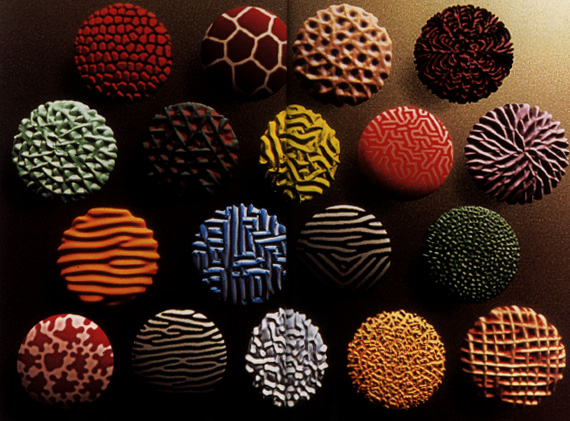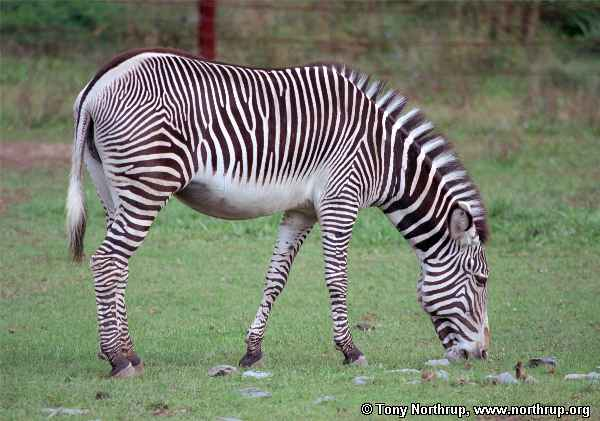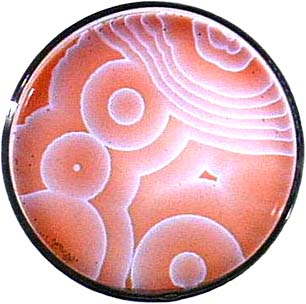1. Consider the Discretized CIMA chemical system in 1-D, and use Matlab and MatCont to obtain global bifurcation diagrams of equilibrium and periodic solutions.
2. Consider another system: reaction-diffusion predator-prey system for the similar questions
3. Consider the impact of spatial heterogeneity (synchronization of coupled oscillations)
4. Bifurcation in water-limited reaction-diffusion-advection systems (traveling band solutions)
5. Higher dimensional reaction-diffusion systems (2-D: planar lattice)

Student research will be partially supported by National Science Foundation grants DMS-0703532(CSUMS: Computational Science Training for Undergraduates in the Mathematical Sciences), and  EF-0436318 (UBM: Undergraduate Research in Metapopulation Ecology). Please contact me for applications of the support (or Dr. Li for CSUMS support, or Dr. Dan Cristo for UBM support). You can work on some problems from topics above, or work on some topic of your own choice, such as developing and analysing a mathematical model in your area of interest. For the mathematical background of the research, you should have completed either Math 345 Mathematical Models in Biology or Math 302 Differential Equations when you start the project. Fo some projects, you can take Math 490 PDE and Mathematical Biology  as a training course on the basic knowledge on reaction-diffusion equations, and Math 413/414 Numerical Analysis for numerical helps.

(an author with * is undergraduate student, and with ** is a W&M undergraduate)

1. Bifurcation for population models with nonlinear diffusion
(spring 2004, supported by NSF DMS-0314736)
Young He Lee, Lena Sherbakov, Jackie Taber
Bifurcation Diagrams of Population Models with Nonlinear Diffusion (Young He Lee**, Lena Sherbakov**, Jackie Taber**, Junping Shi)
(Journal of Computational and Applied Mathematics, Vol. 194, no. 2, 357--367, 2006.)
Lena Sherbakov went to University of Washington for PhD in Applied Math, Jackie Taber stayed in W&M for COR master program.

2. Models of Water-limitted ecosystems (spring 2006, supported by NSF DMS-0314736)
Kristina Little
Cross-diffusion induced instability and stability in reaction-diffusion systems (Junping Shi, Zhifu Xie, Kristina Little**) (submitted)
Kristina Little went to University of Virginia for PhD in Bioengineering.

3. 2-D radial solutions of Fisher equation (spring 2006)
Fumie Hirata
An approximate solution to higher dimensional Fisher equation (Fumie Hirata*, Junping Shi)(preprint)
Fumie Hirata was an exchange master student from Keio University, Japan.

4. Numerical calculation of radial solutions of coupled Schrodinger equations background material (summer 2008, supported by CSUMS:DMS-0703532)
Michael Essman
Bifurcation diagrams of coupled Schr\"odinger equations (Michael Essman**, Junping Shi) (submitted)
presentation in GMU-WM 2009 Spring Workshop (3/21/2009)
poster presentation in 2010 Joint Mathematics Meeting (Jan/2010)
W&M Honor thesis: 2009-2010
Michael Essman is a NASA research assistant in summer 2009 in Hampton, VA.

5. Three pool model of Calcium signaling (summer 2008, supported by UBM:EF-0436318 and CSUMS:DMS-0703532)
Three pool model of Calcium signaling (Daniel Hariprasad**, Meagan McNulty, Junping Shi, Paul Tian) (submitted)
presentation in GMU-WM 2009 Spring Workshop (3/21/2009)
W&M Honor thesis: April 2009
Daniel Hariprasad is now in University Arizona for PhD in Applied Mathematics (2009).

6. Bifurcations in CIMA reaction model (summer 2008)
Jiayin Jin
Bifurcations of Patterned Solutions in Diffusive Lengyel-Epstein System of CIMA Chemical Reaction (Jiayin Jin*, Junping Shi, Junjie Wei, Fengqi Yi) (submitted)
presentation in 2008 Harbin Differential Equation Conference (Dec/2008)
Jiayin Jin is now in Michigan State University for PhD in Mathematics (2009).

7. Alternating stable states in oyster population model (summer 2009, jointly with Rom Lipcius, Jian Shen, Paul Tian, supported by UBM:EF-0436318 and CSUMS:DMS-0703532)
Will Jordan-Cooley
poster presentation in 2010 Joint Mathematics Meeting (Jan/2010)
presentation in GMU-WM 2010 spring Workshop (4/17/2010),
W&M Honor thesis: 2009-2010

8. Bifurcation of Oscillatory and Spatial Patterns in a Diffusive Predator-Prey System (summer 2009, supported by Charles Center BioMath Fellowship)
Yuanyuan Liu
presentation in GMU-WM 2010 spring Workshop (4/17/2010),
W&M Honor thesis: 2009-2010

9. (summer 2010, supported by Charles Center BioMath Fellowship) Ruoyan Sun

10. (summer 2010, supported by CSUMS:DMS-0703532) Matt Becker

11. (summer 2010, supported by CSUMS:DMS-0703532) Tim Becker

Softwares

Matlab, Pplane(a Matlab package for phase plane), MatCont (a Matlab package for bifurcation/continuation), AUTO, XPPAUT,

### The courses I taught on applied mathematics

Math 490 PDE and Mathematical Biology    (Spring 2002, Spring 2004, Spring 2006, Spring 2009) Lecture notes of Math 490
Math 441 Applied Mathematics (Fall 2002)

Math 345
Mathematical Models in Biology  (Fall 2006) (or Math 410 before 2004Mathematical Models in Biology    (Fall 2001)

My publications

The powerpoint file of an undergraduate leecture on Pattern formation in mathematical biology (partly in Chinese)

#### Some population science books related to mathematical biology and differential equations

Life's Other Secret: The New Mathematics of the Living World (Paperback)
by Ian Stewart

Mathematics in Nature : Modeling Patterns in the Natural World (Hardcover)